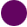SEARCH HOMEMath Central Quandaries & QueriesQuestion from Mario, a parent: Write a division sentence modeled by an array that has 2 more rows than the number in each row.Mario,

I decided to put 3 items in each row of my array, you can choose any positive integer you want. Since the array is to have 2 more rows than the number in each row my array will have 3 + 2 = 5 rows.So I might say that 15 divided by 3 is 5 or 15 divided by 5 is 3.

PennyMath Central is supported by the University of Regina and The Pacific Institute for the Mathematical Sciences.